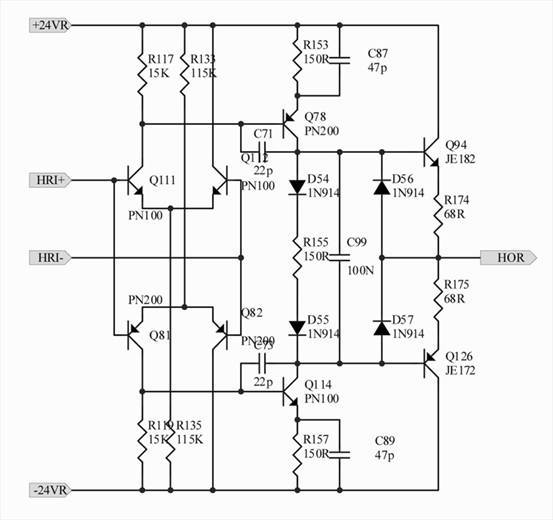# Discrete Differential Amplifier

• oteggis
In summary, the conversation discusses calculating the open loop output impedance and differential gain at 1kHz for a circuit with an input stage, second stage, and output stage. The approach involves disregarding certain parameters and simplifying the circuit, and after correcting some errors, the open loop gain is calculated to be approximately 3281.3 or 70.32dB with a load of 600 ohms.

## Homework Statement

Calculating the open loop output Impedance at 1kHz and the open loop differential gain## The Attempt at a Solution

Here is the approach.

INPUT STAGE: Q111 and Q112

Av1 = Rtot/2re, where Rtot is the total resistance of R117 and the input resistance of Q78 which is Rin.
So the gain Av = Rtot/500 ohms.

If hFE for Q78 is 250,

Rin = hFE*(R115+re) = 250(155) = 38750 ohms.
So Rtot = R117||Rin = 14441ohms = 14000 ohms.

Using this value the gain G1 = 14000/500 = 28.

If the load is 10 ohms

hFE for Q78 and Q94 are 250 and 100 respectively.

Load impedance RL= (250)(100)(600 ohms) = 15000000 ohms.

SECOND STAGE: Q78

Output resistance of Q78:
Using Early voltage of BC557 is 50V,

ro = (VA + VCE)/IC.

ro = 14360 ohms.
Early Effect Resistance Rout = 14360 ohms

The early effect resistance and load impedance are parallel.
So Rtot2 = RL|| Rout = 14346 ohms.

Gains at stage 2: G2 = 14346/155 = 93.

OUTPUT STAGE: Q94 and Q126

For Q94, IE = 10.3mA = 10mA
So re = VT/IE - 25mV/10mA = 2.5 ohms

Rtot3 = R174 + re = 68 ohms + 2.5 ohms = 70.5 ohms

Both halves are in parallel, So

Output Impedance Zout = 70.5 ohms || 70.5 ohms = 35.25 ohms = 35 ohms

Gain in the output stage G3 = RL/(RL + Zout) = 600 ohms/(600 ohms + 35 ohms)= 0.95

Thus open loop gain G = G1*G2*G3 = (28)(93)(0.95) = 2473.8

Converting to dB : Gain in dB = 20log(2473.8) = 68 dB

I have calculated the open loop gain considering a load of 600 ohms. I ended up with an open loop gain of about 2473.8 which is about 68dB.

Is this feasible?

Last edited by a moderator:
oteggis said:
INPUT STAGE: Q111 and Q112
Av1 = Rtot/2re, where Rtot is the total resistance of R117 and the input resistance of Q78 which is Rin.
So the gain Av = Rtot/500 ohms.
If hFE for Q78 is 250, ...
Disregard beta and re. Beta is infinite and re is zero. This problem is complicated enough without including those two parameters.
I would also disregard the capacitors, hoping that at 1 KHz they are ignorable. But if you're really ambitious, include them.
The circuit is mirror-identical between the npn and pnp input stages so work with the npn only, assuming HRI+ > HRI- so the pnp's are not in the gain stages.

You have the following expression: Rin = hFE*(R115+re) = 250(155) = 38750 ohms.

Where is R115?

Sorry, it is a mistake, I wanted to write R153

I also see this calculation: So Rtot = R117||Rin = 14441ohms = 14000 ohms.

If R117 = 15k and Rin = 38750 ohms, we should have 15000||38750 = 10814 ohms, not 14441 ohms.

You are correct. Using the value Rtot = 10814, the gain at this stage G1 is 22

Making the total gain to be

The open loop Gain G = G1*G2*G3 = (22)(157)(0.95) = 3281.3
Converting to dB: GaindB = 20 log(3281.3) ≈ 70.32dB

Another Mistake, If the load is 10 ohms

The load is 600 ohms and not 10 ohms